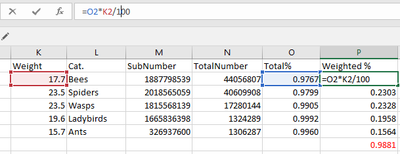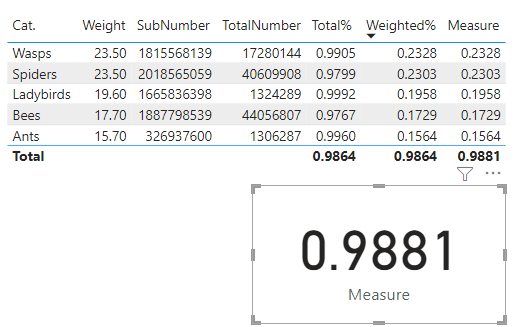cancel
Showing results for
Did you mean:Frequent Visitor

## Summed Category * static weighted value

Hi all, I've been trying to figure this out for days now with no luck online. Hopefully someone can help me.

So I have a data-set of numbers I'd like to sum up by category then another calculation that gets that total category sum and times it by the weighted value of a particular category as below.

I currently have a measure that computes the following "(TotalNumber - SubNumber) / TotalNumber" : to get the "Total %", then I have a calculated column that has an if statment that says(To reference the weighted values),

IF(Availability_Calculations[Avail_Category] = "Spiders" , 23.5,
IF(Availability_Calculations[Avail_Category] = "Wasps", 23.50,
IF(Availability_Calculations[Avail_Category] = "Ants" , 15.70,
IF(Availability_Calculations[Avail_Category] = "Bees" , 17.70,0)))))

Then another column:

[Total %] * [Weighted_Values]

My logic in Excel that I want to replicate but also the red value I want to have in a measure on its own.So my question is, how can I best represent this Excel formula and calculate the total% * the weighted values then following this, show the total % (in red text on the bottom right of the screenshot) So I get my final %?

In Power BI my totals are off. But also, I'd like the percentages to be in a separate measure so I can show it in a card.2 ACCEPTED SOLUTIONSSuper User

@Mike_Infinity2 , It should be table or some group level

a measure

sumx( Availability_Calculations,[Total %] * [Weighted_Values])

or

sumx( values(Availability_Calculations[Avail_Category]) ,calculate([Total %] * max([Weighted_Values]) )  )

!! Microsoft Fabric !!
Microsoft Power BI Learning Resources, 2023 !!
Learn Power BI - Full Course with Dec-2022, with Window, Index, Offset, 100+ Topics !!
Did I answer your question? Mark my post as a solution! Appreciate your Kudos !! Proud to be a Super User! !!Community Support

You can try the following methods.
Initial data:Measure:

``Total% = DIVIDE(SUM('Table'[SubNumber])-SUM('Table'[TotalNumber]),SUM('Table'[SubNumber]))``
``Weighted% = SUM('Table'[Weight])*[Total%]/100``
``Measure = SUMX('Table',[Weighted%])``Is this the result you expect?

Best Regards,

Community Support Team _Charlotte

If this post helps, then please consider Accept it as the solution to help the other members find it more quickly.

3 REPLIES 3Community Support

You can try the following methods.
Initial data:Measure:

``Total% = DIVIDE(SUM('Table'[SubNumber])-SUM('Table'[TotalNumber]),SUM('Table'[SubNumber]))``
``Weighted% = SUM('Table'[Weight])*[Total%]/100``
``Measure = SUMX('Table',[Weighted%])``Is this the result you expect?

Best Regards,

Community Support Team _Charlotte

If this post helps, then please consider Accept it as the solution to help the other members find it more quickly.Frequent Visitor

If I use @amitchandak  calculation of "sumx( values(Availability_Calculations[Avail_Category]) ,calculate([Total %] * max([Weighted_Values]) )  )" it shows up correctly on both the weighted% total and values and if I use @v-zhangti other calculations it works hand in hand for the other sections! Thank you both!Super User

@Mike_Infinity2 , It should be table or some group level

a measure

sumx( Availability_Calculations,[Total %] * [Weighted_Values])

or

sumx( values(Availability_Calculations[Avail_Category]) ,calculate([Total %] * max([Weighted_Values]) )  )

!! Microsoft Fabric !!
Microsoft Power BI Learning Resources, 2023 !!
Learn Power BI - Full Course with Dec-2022, with Window, Index, Offset, 100+ Topics !!
Did I answer your question? Mark my post as a solution! Appreciate your Kudos !! Proud to be a Super User! !!